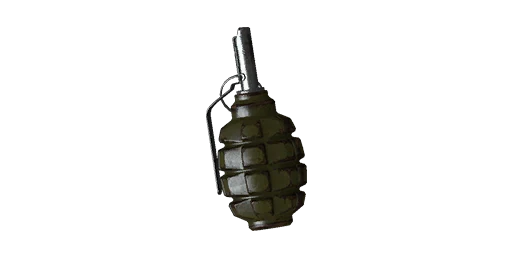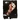For the weapon used in Insurgency: Modern Infantry Combat, see RGD-5 Frag Grenade.

The WF1 (Russian: Фугасный, Fugasnyy; English: Explosive) is a Soviet anti-personnel fragmentation defensive grenade. It is based on the French F1 grenade and contains a 60-gram explosive charge (TNT). The total weight of the grenade with the fuze is about 600 grams.

Due to its shape and its yellow-green color, it is nicknamed the limonka (fem. "lemon").

## Insurgency

{{#infoboxbuilder: | 0:Theme = INS default | 0:CustomModule = | 0:MainImageCaption = | 0:ToggleContentLongerThan = 1500

| 1:Type = Title

```| 1:Value = F1 Frag
```

| 2:Type = MainImage

```| 2:Value =```

| 3:Value =

General information

| 4:Type = Line

```| 4:Label = Inventory slot
| 4:Value = Explosive
```

| 5:Type = Line

```| 5:Label = Weapon class
```

| 7:Type = Line

```| 7:Label = Operators
| 7:Value =Insurgent Forces
```

| 9:Type = Line

```| 9:Label = Console ?
| 9:Value =
```

| 10:Value =

Statistics
Damage

| 11:Type = Line

```| 11:Label = Damage
| 11:Value = 160
```

| 12:Type = Line

```| 12:Label = Explosion radius
```

| 12:Value = 750

| 14:Type = Line

```| 14:Label = Max range ?
| 14:Value =
```

| 15:Type = Line

```| 15:Label = Rate of fire
| 15:Value = 30
```

| 16:Type = Line

```| 16:Label = Fuse time
| 16:Value = 4s
```

| 17:Type = Line

```| 17:Label = Cookable?
| 17:Value = Yes
```

| 18:Type = Line

```| 18:Label = Effect duration
| 18:Value =
```

| 19:Value =

Ammunition

| 22:Type = Line

```| 22:Label = Starting ammo
| 22:Value = 1If equipped in explosive slot and explosive two slot:2 (CR)3 (CC)
```

| 23:Type = Line

```| 23:Label = Reload time
| 23:Value =
```

| 29:Value =

General statistics

| 30:Type = Line

```| 30:Label = Suppression
| 30:Value =
```

| 31:Type = Line

```| 31:Label = Penetration
| 31:Value =
```

| 32:Type = Line

```| 32:Label = Optic magnification
| 32:Value =
```

| 33:Type = Line

```| 33:Label = Supply points ?
| 33:Value = 2```

| 34:Type = Line

```| 34:Label = Weight points ?
| 34:Value = 27/640
```

| 35:Type = Line

```| 35:Label = Weight
| 35:Value = 0.6 kg
```

| 36:Type = Line

```| 36:Label = Length ?
| 36:Value = 21
```

}}

The F1 Frag is a fragmentation grenade featured in Insurgency.

## Insurgency: Sandstorm

{{#infoboxbuilder: | 0:Theme = IS default | 0:CustomModule = | 0:MainImageCaption = | 0:ToggleContentLongerThan = 1500

| 1:Type = Title

```| 1:Value = F1 Frag
```

| 2:Type = MainImage

```| 2:Value = File:IS F1 Frag.png
```

| 3:Value =

General information

| 4:Type = Line

```| 4:Label = Inventory slot
| 4:Value = Explosive
```

| 5:Type = Line

```| 5:Label = Weapon class
```

| 7:Type = Line

```| 7:Label = Operators
| 7:Value =Insurgent Forces
```

| 9:Type = Line

```| 9:Label = Console ?
| 9:Value =
```

| 10:Value =

Statistics
Damage

| 11:Type = Line

```| 11:Label = Damage
| 11:Value =
```

| 12:Type = Line

```| 12:Label = Explosion radius
| 12:Value =
```

| 14:Type = Line

```| 14:Label = Max range ?
| 14:Value =
```

| 15:Type = Line

```| 15:Label = Rate of fire
| 15:Value =
```

| 16:Type = Line

```| 16:Label = Fuse time
| 16:Value =
```

| 17:Type = Line

```| 17:Label = Cookable?
| 17:Value =
```

| 18:Type = Line

```| 18:Label = Effect duration
| 18:Value =
```

| 19:Value =

Ammunition

| 22:Type = Line

```| 22:Label = Starting ammo
| 22:Value = 1 grenade2 (LC)3 (HC)
```

| 23:Type = Line

```| 23:Label = Reload time
| 23:Value =
```

| 29:Value =

General statistics

| 30:Type = Line

```| 30:Label = Suppression
| 30:Value =
```

| 31:Type = Line

```| 31:Label = Penetration
| 31:Value =
```

| 32:Type = Line

```| 32:Label = Optic magnification
| 32:Value =
```

| 33:Type = Line

```| 33:Label = Supply points ?
| 33:Value = 2
```

| 34:Type = Line

```| 34:Label = Weight points ?
| 34:Value =
```

| 35:Type = Line

```| 35:Label = Weight
| 35:Value = 4%
```

| 36:Type = Line

```| 36:Label = Length ?
| 36:Value =
```

}}

"Explosive grenade which detonates into lethal fragments."
— In-game description

The F1 Frag is a fragmentation grenade featured in Insurgency: Sandstorm.

## Trivia

Insurgency

• The M67 and F1 frag grenades are identical in performance.